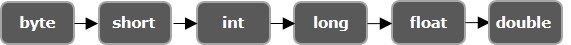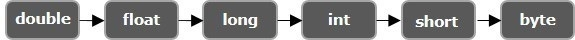# What is type conversion in java?

Java provides various datatypes to store various data values. It provides 7 primitive datatypes (stores single values) as listed below −

• boolean − Stores 1-bit value representing true or, false.
• byte − Stores twos compliment integer up to 8 bits.
• char − Stores a Unicode character value up to 16 bits.
• short − Stores an integer value upto 16 bits.
• int − Stores an integer value upto 32 bits.
• long − Stores an integer value upto 64 bits.
• float − Stores a floating point value upto 32bits.
• double − Stores a floating point value up to 64 bits.

## Type Casting/type conversion

Converting one primitive datatype into another is known as type casting (type conversion) in Java. You can cast the primitive datatypes in two ways namely, Widening and, Narrowing.

Widening − Converting a lower datatype to a higher datatype is known as widening. In this case the casting/conversion is done automatically therefore, it is known as implicit type casting. In this case both datatypes should be compatible with each other.## Example

public class WideningExample {
public static void main(String args[]){
char ch = 'C';
int i = ch;
System.out.println(i);
}
}

## Output

Integer value of the given character: 67

Narrowing − Converting a higher datatype to a lower datatype is known as narrowing. In this case the casting/conversion is not done automatically, you need to convert explicitly using the cast operator “( )” explicitly. Therefore, it is known as explicit type casting. In this case both datatypes need not be compatible with each other.## Example

import java.util.Scanner;
public class NarrowingExample {
public static void main(String args[]){
Scanner sc = new Scanner(System.in);
System.out.println("Enter an integer value: ");
int i = sc.nextInt();
char ch = (char) i;
System.out.println("Character value of the given integer: "+ch);
}
}

## Output

Enter an integer value:
67
Character value of the given integer: C